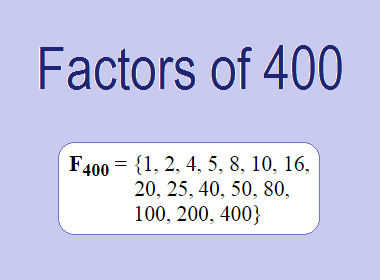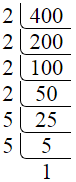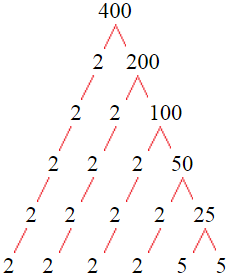# Factors of 400The factors of 400 are 1, 2, 4, 5, 8, 10, 16, 20, 25, 40, 50, 80, 100, 200, and 400 i.e. F400 = {1, 2, 4, 5, 8, 10, 16, 20, 25, 40, 50, 80, 100, 200, 400}. The factors of 400 are all the numbers that can divide 400 without leaving a remainder.

We can check if these numbers are factors of 400 by dividing 400 by each of them. If the result is a whole number, then the number is a factor of 400. Let's do this for each of the numbers listed above:

·        1 is a factor of 400 because 400 divided by 1 is 400.

·        2 is a factor of 400 because 400 divided by 2 is 200.

·        4 is a factor of 400 because 400 divided by 4 is 100.

·        5 is a factor of 400 because 400 divided by 5 is 80.

·        8 is a factor of 400 because 400 divided by 8 is 50.

·        10 is a factor of 400 because 400 divided by 10 is 40.

·        16 is a factor of 400 because 400 divided by 16 is 25.

·        20 is a factor of 400 because 400 divided by 20 is 20.

·        25 is a factor of 400 because 400 divided by 25 is 16.

·        40 is a factor of 400 because 400 divided by 40 is 10.

·        50 is a factor of 400 because 400 divided by 50 is 8.

·        80 is a factor of 400 because 400 divided by 80 is 5.

·        100 is a factor of 400 because 400 divided by 100 is 4.

·        200 is a factor of 400 because 400 divided by 200 is 2.

·        400 is a factor of 400 because 400 divided by 400 is 1.

## How to Find Factors of 400?

1 and the number itself are the factors of every number. So, 1 and 400 are two factors of 400. To find the other factors of 400, we can start by dividing 400 by the numbers between 1 and 400. If we divide 400 by 2, we get a remainder of 0. Therefore, 2 is a factor of 400. If we divide 400 by 3, we get a remainder of 1. Therefore, 3 is not a factor of 400.

Next, we can check if 4 is a factor of 400. If we divide 400 by 4, we get a remainder of 0. Therefore, 4 is also a factor of 400. We can continue this process for all the possible factors of 400.

Through this process, we can find that the factors of 400 are 1, 2, 4, 5, 8, 10, 16, 20, 25, 40, 50, 80, 100, 200, and 400. These are the only numbers that can divide 400 without leaving a remainder.

********************

********************

## Properties of the Factors of 400

The factors of 400 have some interesting properties. One of the properties is that the sum of the factors of 400 is equal to 961. We can see this by adding all the factors of 400 together:

1 + 2 + 4 + 5 + 8 + 10 + 16 + 20 + 25 + 40 + 50 + 80 + 100 + 200 + 400 = 961

Another property of the factors of 400 is that the prime factors of 400 are 2, and 5 only.

## Applications of the Factors of 400

The factors of 400 have several applications in mathematics. One of the applications is in finding the highest common factor (HCF) of two or more numbers. The HCF is the largest factor that two or more numbers have in common. For example, to find the HCF of 400 and 150, we need to find the factors of both numbers and identify the largest factor they have in common. The factors of 400 are 1, 2, 4, 5, 8, 10, 16, 20, 25, 40, 50, 80, 100, 200, and 400. The factors of 150 are 1, 2, 3, 5, 6, 10, 15, 25, 30, 50, 75, and 150. The largest factor that they have in common is 50. Therefore, the HCF of 400 and 150 is 50.

Another application of the factors of 400 is in prime factorization. Prime factorization is the process of expressing a number as the product of its prime factors. The prime factors of 400 are 2, and 5 since these are the only prime numbers that can divide 400 without leaving a remainder. Therefore, we can express 400 as:

400 = 2 × 2 × 2 × 2 × 5 × 5

We can do prime factorization by division and factor tree method also. Here is the prime factorization of 400 by division method,400 = 2 × 2 × 2 × 2 × 5 × 5

Here is the prime factorization of 400 by the factor tree method,400 = 2 × 2 × 2 × 2 × 5 × 5

## Conclusion

The factors of 400 are the numbers that can divide 400 without leaving a remainder. The factors of 400 are 1, 2, 4, 5, 8, 10, 16, 20, 25, 40, 50, 80, 100, 200, and 400. The factors of 400 have some interesting properties, such as having a sum of 961. The factors of 400 have several applications in mathematics, such as finding the highest common factor and prime factorization.